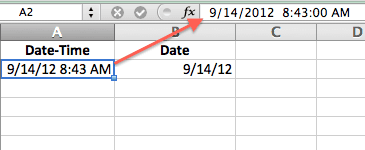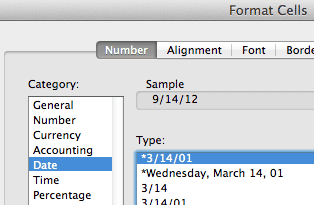# Extract a Date from a Date-Time Number in Excel

I recently saw a spreadsheet with the following function =LEFT(A2,8) where cell A2 was equal to 08/06/12 12:23 PM. The user was trying to extract the Date from the Date-Time value. The problem for me was that the LEFT function returns a “text” value. Excel is good at recognizing text values that look like dates, as Dates, but why not use a formula that returns a numeric value?

My reflex was to enter the following formula =DATE(YEAR(A2),MONTH(A2),DAY(A2)) and get a proper Date value. You can tell the difference because, with no cell formatting, a Text value is left-justified in the cell, and a Date value is right-justified in the cell, meaning that it’s a number.

I got to thinking later that there’s a simpler formula to extract the Date from a Date-Time value, which is =INT(A2). After you enter this formula, the cell formatting needs to be changed to a Date format.

### Why Does the INT Function Extract a Date?

All Dates, Times, and Date-Times are know as serial numbers in Excel. You don’t normally see the underlying serial number. A Date-Time value like 9/14/2012 8:43 AM looks like that in the cell AND in the formula bar.Date Time shown in Cell and Formula Bar

Only when you change the cell formatting to General do you see the Date-Time serial number. The integer portion is the date serial number and the fractional part is the time serial number.Serial Date Time

This is why the INT function will work to extract a Date from a Date-Time value. The integer value is a date serial number. The trick is to change the cell formatting to a Date format so that Excel will show you the Date.Format Cells Date